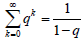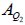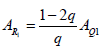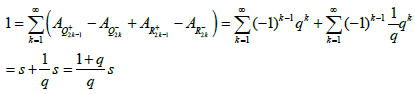## Research and Reports on Mathematics

All submissions of the EM system will be redirected to Online Manuscript Submission System. Authors are requested to submit articles directly to Online Manuscript Submission System of respective journal.

Short Communication, Res Rep Math Vol: 2 Issue: 3

# Geometric Proof of the Sum of Geometric Series

Korus P*

Department of Mathematics, Juhasz Gyula Faculty of Education University of Szeged, Hungary

*Corresponding Author : Korus P
Department of Mathematics, Juhasz Gyula Faculty of Education University of Szeged, Hattyas utca 10, H-6725 Szeged, Hungary
E-mail: korpet@jgypk.u-szeged.hu

Received: March 28, 2018 Accepted: July 10, 2018 Published: September 8, 2018

Citation: Korus P (2018) Geometric Proof of the Sum of Geometric Series. Res Rep Math 2:3

## Abstract

The well-known formula for the sum of the geometric series is For arbitrary -1<q<1. Among analytic proofs, geometric proofs were also given for this formula, see , mostly for 0<q<1. Now we prove that for any 0<q<1

## Introduction

The well-known formula for the sum of the geometric series isFor arbitrary -1<q<1. Among analytic proofs, geometric proofs were also given for this formula, see , mostly for 0<q<1. Now we prove that for any 0<q<1holds in the ‘Positive case’ andin the ‘Alternating case’.

## Positive case

As in Figure 1, we do the following process.

Step 1: Take a unit square S1 and take rectangles Q1 with area= q and Q2 with= q2. We also take ‘adjunct’ rectangles R1 withand R2 withWe get a remaining square S2 of side length q.

Step 2: We repeat the actions of the previous step for S2, but we reduce the rectangles by a scale factor of q. Then we get Q3; Q4; R3; R4 withand a remaining square S3 of side length q2.

General step k: We repeat the actions of the previous step for Sk, but we reduce the rectangles by a scale factor of q.

We get that the area of unit square S1 isHenceAlternating case

As in Figure 2, we do the following process.

Step 1: Take a unit square S and take rectangles Q1+ with AQ1+ = q and Q2 with AQ2- = q2. We also take ‘adjunct’ square R1+ of side length 1 withand rectangle R1- of side lengths q and 1 with(Plus and minus signs indicate the signs of the areas of rectangles within the final sum.)

Step 2: We repeat the actions of the previous step for square Q2 of side length q, but we reduce the rectangles by a scale factor of q. Then we getwith,General step k: We repeat the actions of the previous step for Q2k-2 , but we reduce the rectangles by a scale factor of q.

We get that the area of unit squareisHence## References